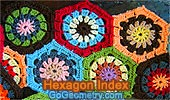# Geometry: Hexagons, Theorems and Problems - Table of Content 3Sangaku Problem (An Old Japanese Theorem). Inradii, Carnot's theorem.

Proposed Problem 61: Triangle, Trisection of Sides

Proposed Problem 122. Marion Walter's Theorem. Area of triangle and Hexagon, Trisection of Sides.

Proposed Problem 125. Area of Triangle, Star, Trisection of Sides.

Kurschak's Tile and Theorem. Jozsef Kurschak (Hungary, 1864-1933) An elegant and a purely geometric way of finding the area of a regular dodecagon.
A regular dodecagon inscribed in a unit circle has area 3.

Go to Page: Previous | 1 | 2 | 3 | 4 | 5 | Next